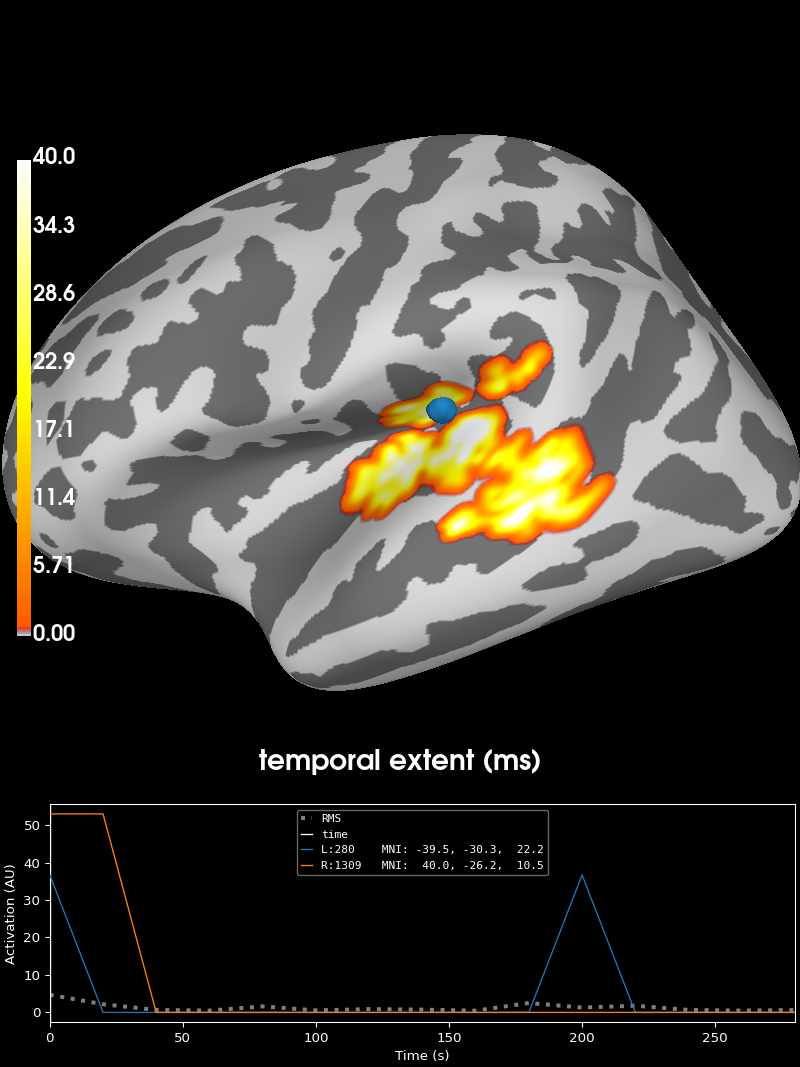# 2 samples permutation test on source data with spatio-temporal clustering#

Tests if the source space data are significantly different between 2 groups of subjects (simulated here using one subject’s data). The multiple comparisons problem is addressed with a cluster-level permutation test across space and time.

```# Authors: Alexandre Gramfort <alexandre.gramfort@inria.fr>
#          Eric Larson <larson.eric.d@gmail.com>
```
```import numpy as np
from scipy import stats as stats

import mne
from mne.datasets import sample
from mne.stats import spatio_temporal_cluster_test, summarize_clusters_stc

print(__doc__)
```

## Set parameters#

```data_path = sample.data_path()
meg_path = data_path / "MEG" / "sample"
stc_fname = meg_path / "sample_audvis-meg-lh.stc"
subjects_dir = data_path / "subjects"
src_fname = subjects_dir / "fsaverage" / "bem" / "fsaverage-ico-5-src.fif"

# Load stc to in common cortical space (fsaverage)

# Read the source space we are morphing to
fsave_vertices = [s["vertno"] for s in src]
morph = mne.compute_source_morph(
stc,
"sample",
"fsaverage",
spacing=fsave_vertices,
smooth=20,
subjects_dir=subjects_dir,
)
stc = morph.apply(stc)
n_vertices_fsave, n_times = stc.data.shape
tstep = stc.tstep * 1000  # convert to milliseconds

n_subjects1, n_subjects2 = 6, 7
print("Simulating data for %d and %d subjects." % (n_subjects1, n_subjects2))

#    Let's make sure our results replicate, so set the seed.
np.random.seed(0)
X1 = np.random.randn(n_vertices_fsave, n_times, n_subjects1) * 10
X2 = np.random.randn(n_vertices_fsave, n_times, n_subjects2) * 10
X1[:, :, :] += stc.data[:, :, np.newaxis]
# make the activity bigger for the second set of subjects
X2[:, :, :] += 3 * stc.data[:, :, np.newaxis]

#    We want to compare the overall activity levels for each subject
X1 = np.abs(X1)  # only magnitude
X2 = np.abs(X2)  # only magnitude
```
```    Reading a source space...
[done]
[done]
surface source space present ...
Computing morph matrix...
20 smooth iterations done.
20 smooth iterations done.
[done]
[done]
Simulating data for 6 and 7 subjects.
```

## Compute statistic#

To use an algorithm optimized for spatio-temporal clustering, we just pass the spatial adjacency matrix (instead of spatio-temporal)

```print("Computing adjacency.")

#    Note that X needs to be a list of multi-dimensional array of shape
#    samples (subjects_k) × time × space, so we permute dimensions
X1 = np.transpose(X1, [2, 1, 0])
X2 = np.transpose(X2, [2, 1, 0])
X = [X1, X2]

# Now let's actually do the clustering. This can take a long time...
# Here we set the threshold quite high to reduce computation,
# and use a very low number of permutations for the same reason.
n_permutations = 50
p_threshold = 0.001
f_threshold = stats.distributions.f.ppf(
1.0 - p_threshold / 2.0, n_subjects1 - 1, n_subjects2 - 1
)
print("Clustering.")
F_obs, clusters, cluster_p_values, H0 = clu = spatio_temporal_cluster_test(
X,
n_jobs=None,
n_permutations=n_permutations,
threshold=f_threshold,
buffer_size=None,
)
#    Now select the clusters that are sig. at p < 0.05 (note that this value
#    is multiple-comparisons corrected).
good_cluster_inds = np.where(cluster_p_values < 0.05)
```
```Computing adjacency.
-- number of adjacent vertices : 20484
Clustering.
stat_fun(H1): min=0.000000 max=303.632172
Running initial clustering …
Found 361 clusters

0%|          | Permuting : 0/49 [00:00<?,       ?it/s]
2%|▏         | Permuting : 1/49 [00:00<00:03,   14.76it/s]
6%|▌         | Permuting : 3/49 [00:00<00:01,   30.05it/s]
8%|▊         | Permuting : 4/49 [00:00<00:01,   29.92it/s]
12%|█▏        | Permuting : 6/49 [00:00<00:01,   36.32it/s]
14%|█▍        | Permuting : 7/49 [00:00<00:01,   35.06it/s]
16%|█▋        | Permuting : 8/49 [00:00<00:01,   34.17it/s]
20%|██        | Permuting : 10/49 [00:00<00:01,   37.87it/s]
22%|██▏       | Permuting : 11/49 [00:00<00:01,   36.76it/s]
27%|██▋       | Permuting : 13/49 [00:00<00:00,   39.55it/s]
29%|██▊       | Permuting : 14/49 [00:00<00:00,   38.41it/s]
31%|███       | Permuting : 15/49 [00:00<00:00,   37.45it/s]
35%|███▍      | Permuting : 17/49 [00:00<00:00,   39.67it/s]
37%|███▋      | Permuting : 18/49 [00:00<00:00,   38.69it/s]
41%|████      | Permuting : 20/49 [00:00<00:00,   40.59it/s]
43%|████▎     | Permuting : 21/49 [00:00<00:00,   39.61it/s]
47%|████▋     | Permuting : 23/49 [00:00<00:00,   41.29it/s]
49%|████▉     | Permuting : 24/49 [00:00<00:00,   40.32it/s]
51%|█████     | Permuting : 25/49 [00:00<00:00,   39.46it/s]
55%|█████▌    | Permuting : 27/49 [00:00<00:00,   40.99it/s]
57%|█████▋    | Permuting : 28/49 [00:00<00:00,   40.13it/s]
61%|██████    | Permuting : 30/49 [00:00<00:00,   41.54it/s]
63%|██████▎   | Permuting : 31/49 [00:00<00:00,   40.68it/s]
67%|██████▋   | Permuting : 33/49 [00:00<00:00,   41.98it/s]
69%|██████▉   | Permuting : 34/49 [00:00<00:00,   41.12it/s]
71%|███████▏  | Permuting : 35/49 [00:00<00:00,   40.34it/s]
76%|███████▌  | Permuting : 37/49 [00:00<00:00,   41.60it/s]
78%|███████▊  | Permuting : 38/49 [00:00<00:00,   40.81it/s]
82%|████████▏ | Permuting : 40/49 [00:00<00:00,   42.00it/s]
84%|████████▎ | Permuting : 41/49 [00:01<00:00,   41.21it/s]
88%|████████▊ | Permuting : 43/49 [00:01<00:00,   42.34it/s]
90%|████████▉ | Permuting : 44/49 [00:01<00:00,   41.56it/s]
92%|█████████▏| Permuting : 45/49 [00:01<00:00,   40.83it/s]
96%|█████████▌| Permuting : 47/49 [00:01<00:00,   41.93it/s]
98%|█████████▊| Permuting : 48/49 [00:01<00:00,   41.19it/s]
100%|██████████| Permuting : 49/49 [00:01<00:00,   41.16it/s]
```

## Visualize the clusters#

```print("Visualizing clusters.")

#    Now let's build a convenient representation of each cluster, where each
#    cluster becomes a "time point" in the SourceEstimate
fsave_vertices = [np.arange(10242), np.arange(10242)]
stc_all_cluster_vis = summarize_clusters_stc(
clu, tstep=tstep, vertices=fsave_vertices, subject="fsaverage"
)

#    Let's actually plot the first "time point" in the SourceEstimate, which
#    shows all the clusters, weighted by duration

# blue blobs are for condition A != condition B
brain = stc_all_cluster_vis.plot(
"fsaverage",
hemi="both",
views="lateral",
subjects_dir=subjects_dir,
time_label="temporal extent (ms)",
clim=dict(kind="value", lims=[0, 1, 40]),
)
``````Visualizing clusters.
```

Total running time of the script: (0 minutes 12.365 seconds)

Estimated memory usage: 191 MB

Gallery generated by Sphinx-Gallery